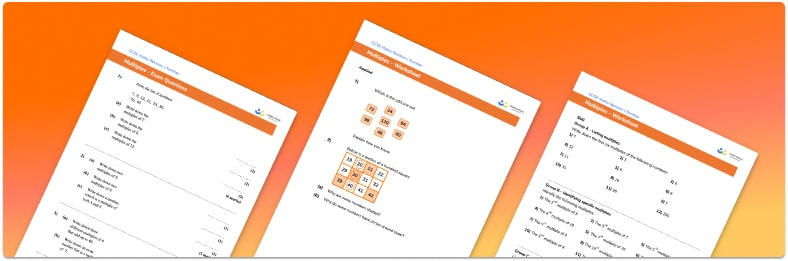# Multiples Worksheet• Section 1 of the multiples worksheet contains 20+ skills-based multiples questions, in 3 groups to support differentiation
• Section 2 contains 3 applied multiples questions with a mix of word problems and deeper problem solving questions
• Section 3 contains 3 foundation and higher level GCSE exam style multiples questions
• Answers and a mark scheme for all multiples questions are provided
• Questions follow variation theory with plenty of opportunities for students to work independently at their own level
• All questions and maths resources created by fully qualified expert secondary maths teachers
• Suitable for GCSE maths revision for AQA, OCR and Edexcel exam boards

• This field is for validation purposes and should be left unchanged.

You can unsubscribe at any time (each email we send will contain an easy way to unsubscribe). To find out more about how we use your data, see our privacy policy.

### Multiplies at a glance

The multiples of a whole number (an integer) are the values in the times table for that number. For example, the multiples of 5 are 5, 10, 15, 20, 25, …. etc. To identify multiples of a number, we look for numbers in the times table

The lowest common multiple (least common multiple/lcm) of two numbers is the smallest value that is a multiple of both numbers. To find the lowest common multiple of two numbers we can either list the multiples of the given numbers until we find a common multiple or find the prime factors of each number and use a Venn diagram to compare the prime factors. To find the LCM from a Venn diagram, we multiply together every number on the Venn diagram.

Looking forward, students can then progress to additional number worksheets, for example a multiplying and dividing decimals worksheet or an order of operations worksheet.For more teaching and learning support on Number our GCSE maths lessons provide step by step support for all GCSE maths concepts.

## Do you have KS4 students who need more focused attention to succeed at GCSE?There will be students in your class who require individual attention to help them succeed in their maths GCSEs. In a class of 30, it’s not always easy to provide.

Help your students feel confident with exam-style questions and the strategies they’ll need to answer them correctly with our dedicated GCSE maths revision programme.

Lessons are selected to provide support where each student needs it most, and specially-trained GCSE maths tutors adapt the pitch and pace of each lesson. This ensures a personalised revision programme that raises grades and boosts confidence.

Find out more